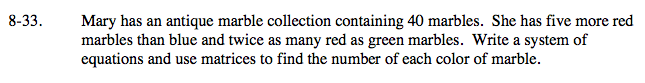Home > A2C > Chapter 8 > Lesson 8.1.2 > Problem8-33

8-33.

Mary has an antique marble collection containing 40 marbles. She has five more red marbles than blue and twice as many red as green marbles. Write a system of equations and use matrices to find the number of each color of marble. Homework Help ✎Let b = the number of blue marbles, r = the number of red marbles,
and g = the number of green marbles.

b + 5 = r
2g = r
r + g + b = 40

Solve the system of equations using a combination of substitution and elimination.

$$\left[ {\begin {array}{ccc}-1&0&1\\-1&2&0\\1&1&1\end{array}}\right]$ \cdot $\left[{\begin{array}{c}\textit{r}\\\textit{g}\\\textit{b}\end{array}}\right]$=$\left[{\begin{array}{c}-5\\0\\40\end{array}}\right]$$